# Concise Selina Solutions for Class 9 Maths Chapter 28 - Distance Formula

The Selina Solutions for the questions given in Chapter 28, Distance Formula, of the Class 9 Selina textbooks are available here. In this chapter students learn about the topic of Distance Formula as well as the method of finding the distance between two points. Students can easily score full marks in the exams by solving all the questions present in the Selina textbook.

The Class 9 Selina Solutions Maths is very easy to understand. These solutions cover all the exercise questions included in the book and are according to the syllabus prescribed by the ICSE or CISCE. Here, the PDF of the Class 9 Maths Chapter 28 Selina Solutions is available, which can be downloaded as well as viewed online. Students can also avail the Selina Solutions and download it for free to practice offline as well.

## Download PDF of Selina Solutions for Class 9 Maths Chapter 28:-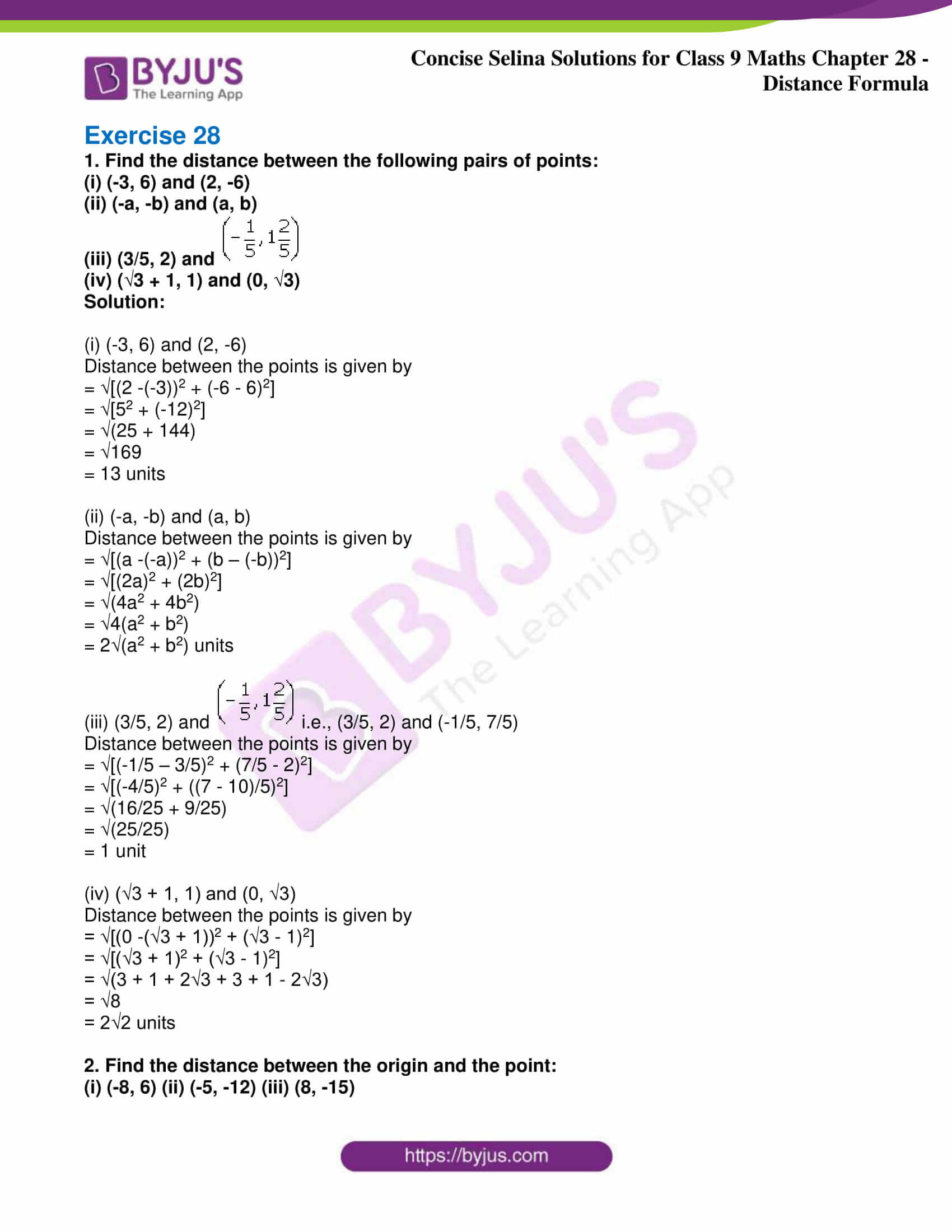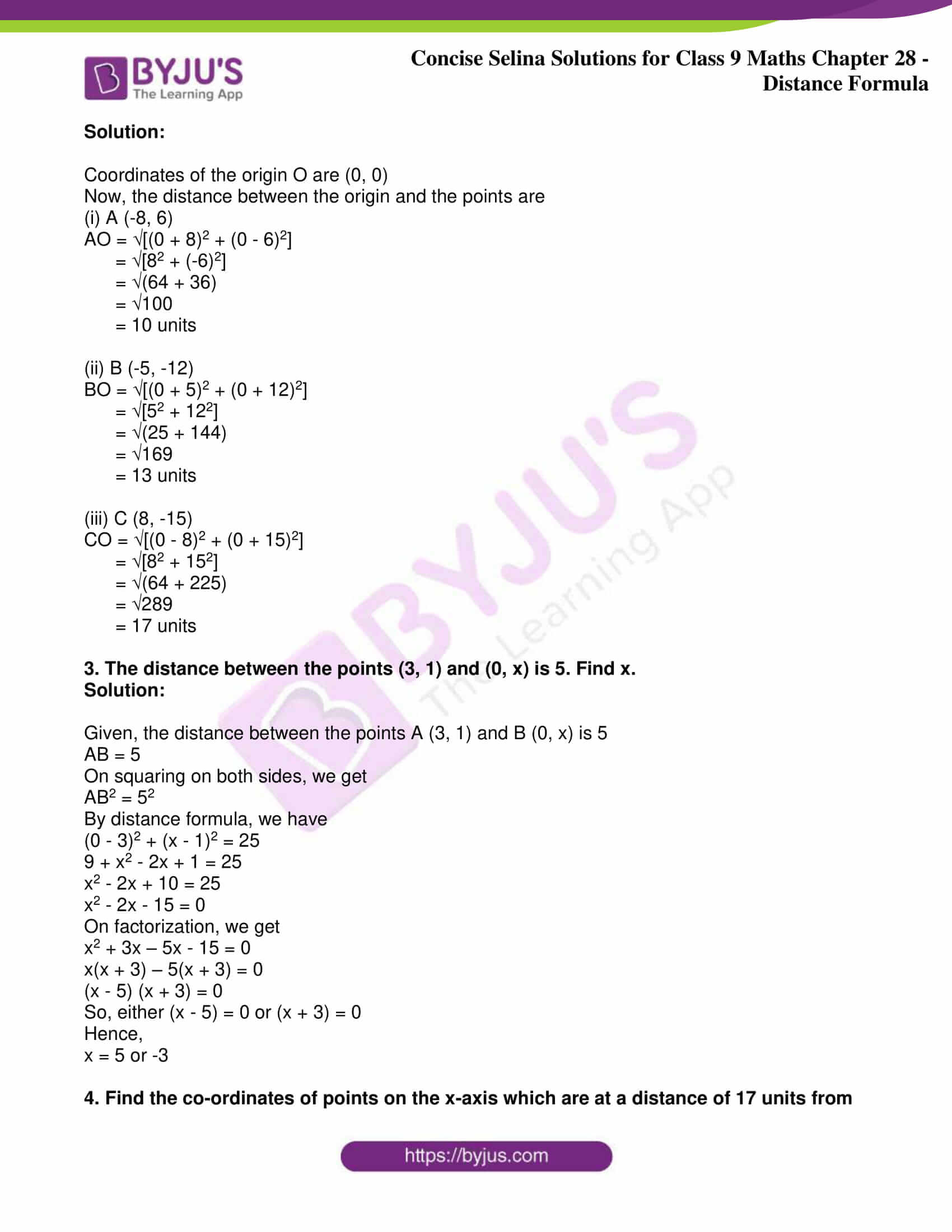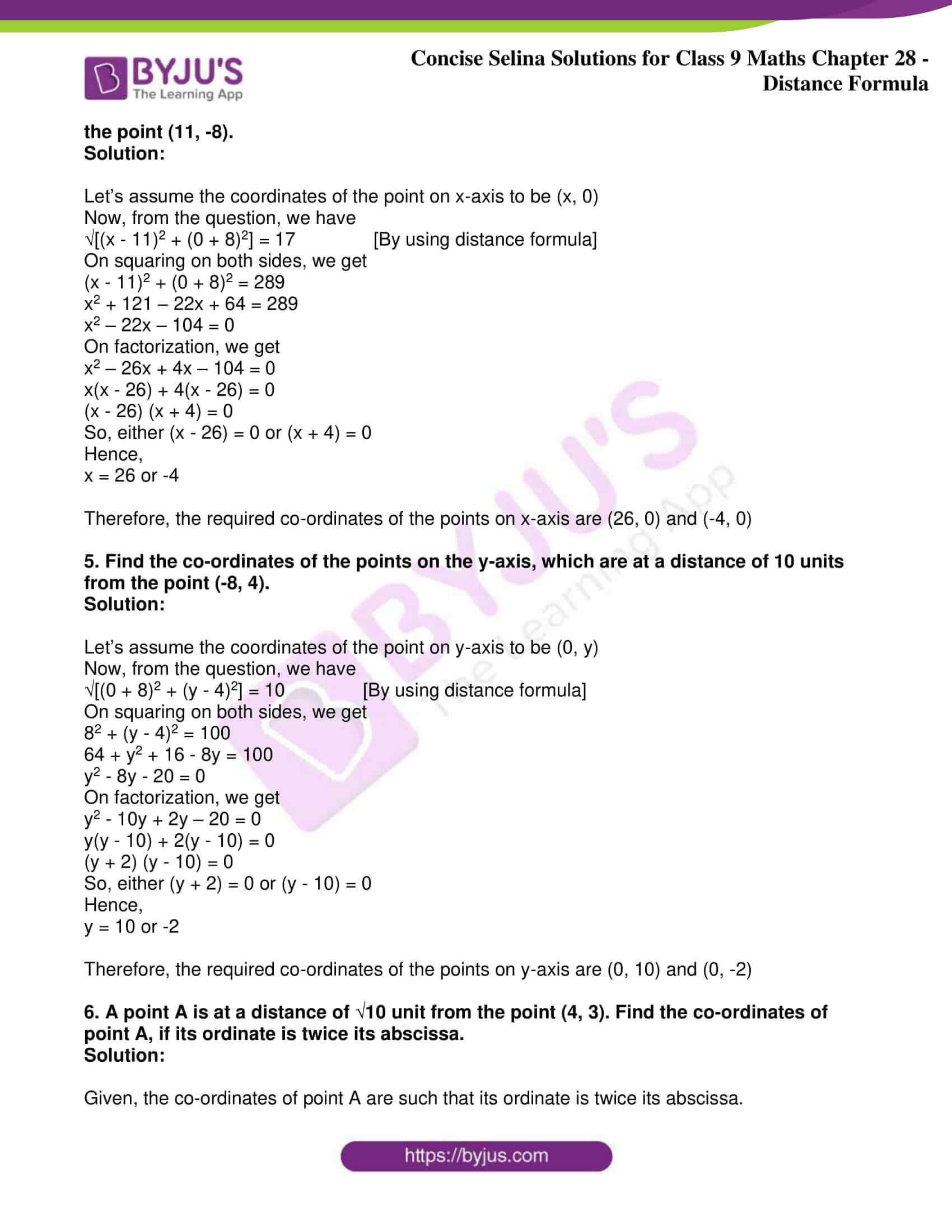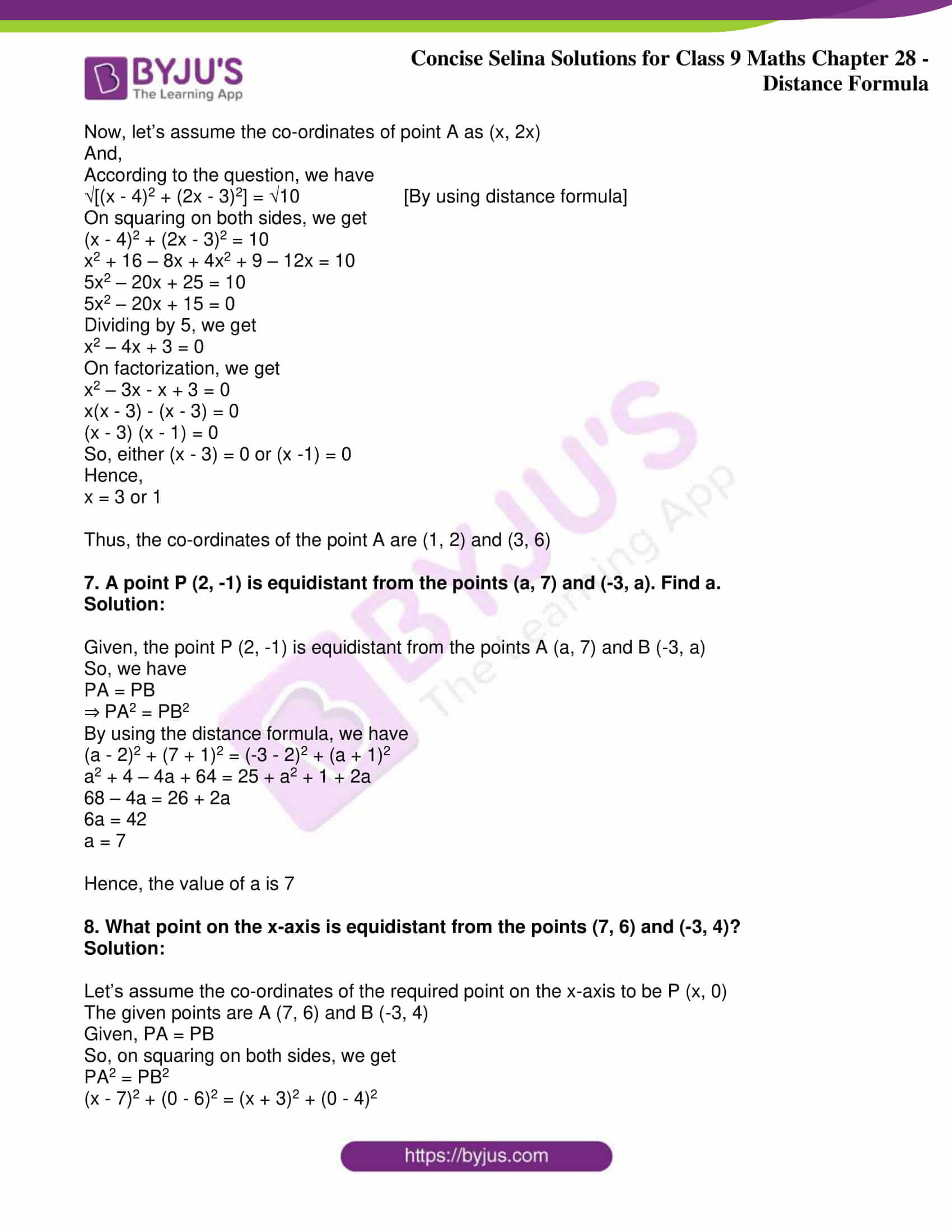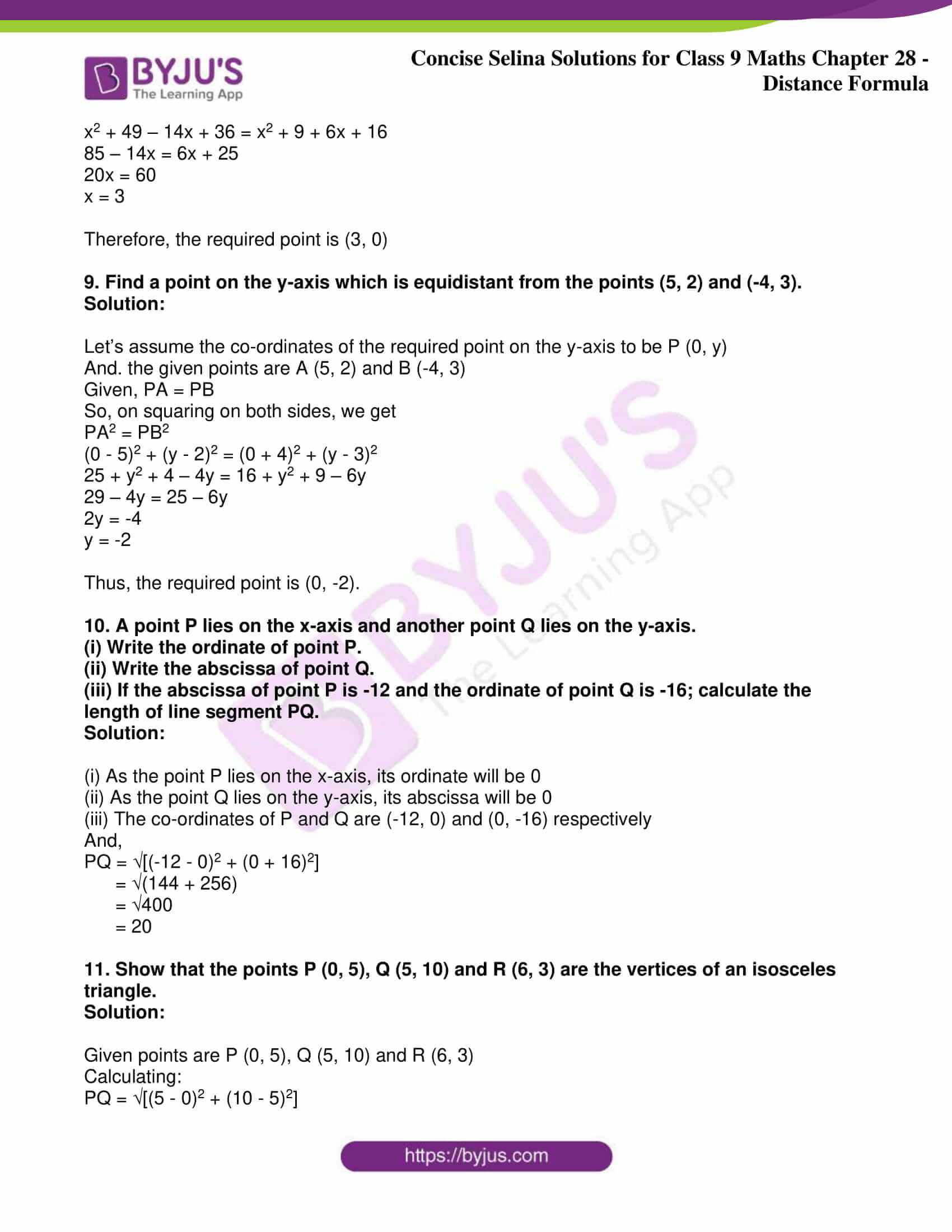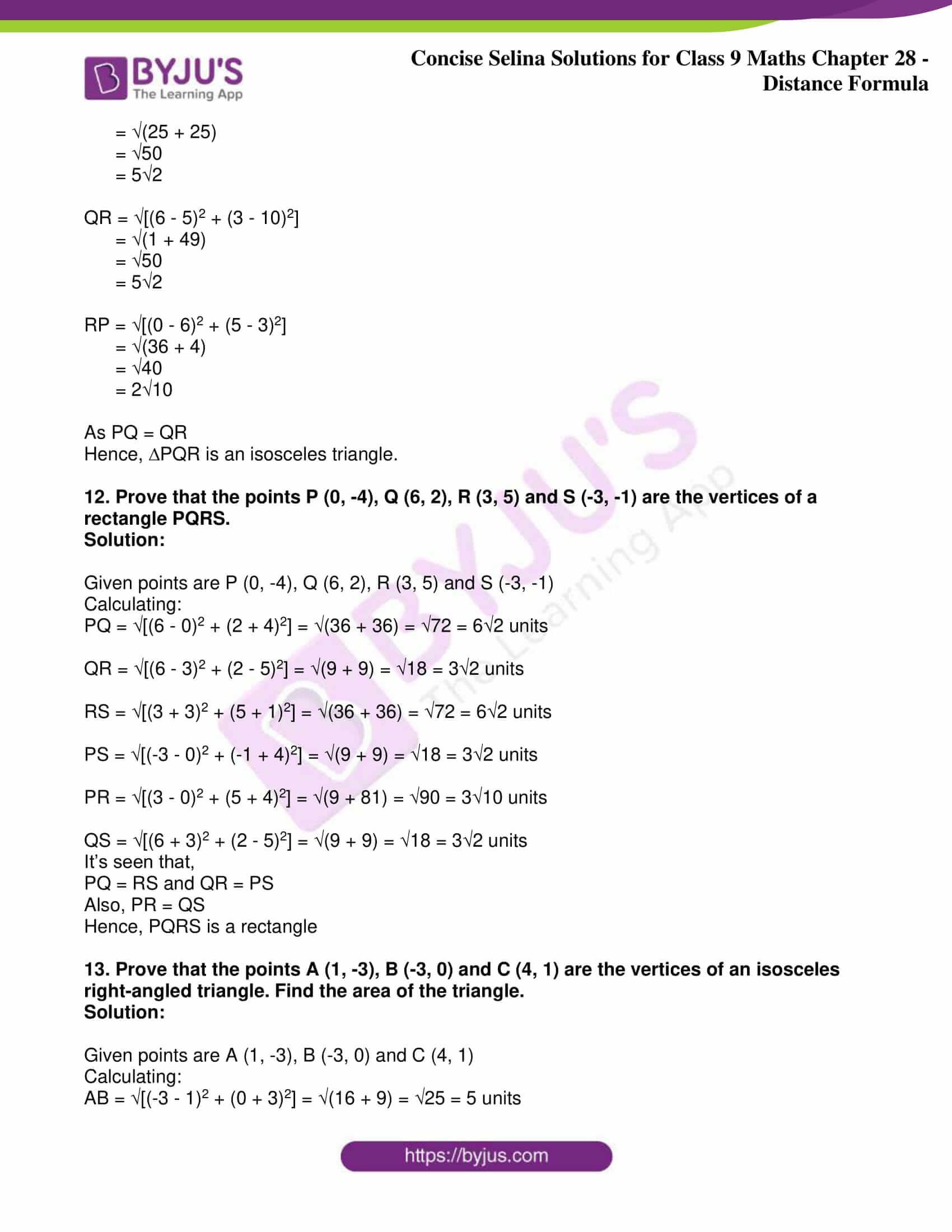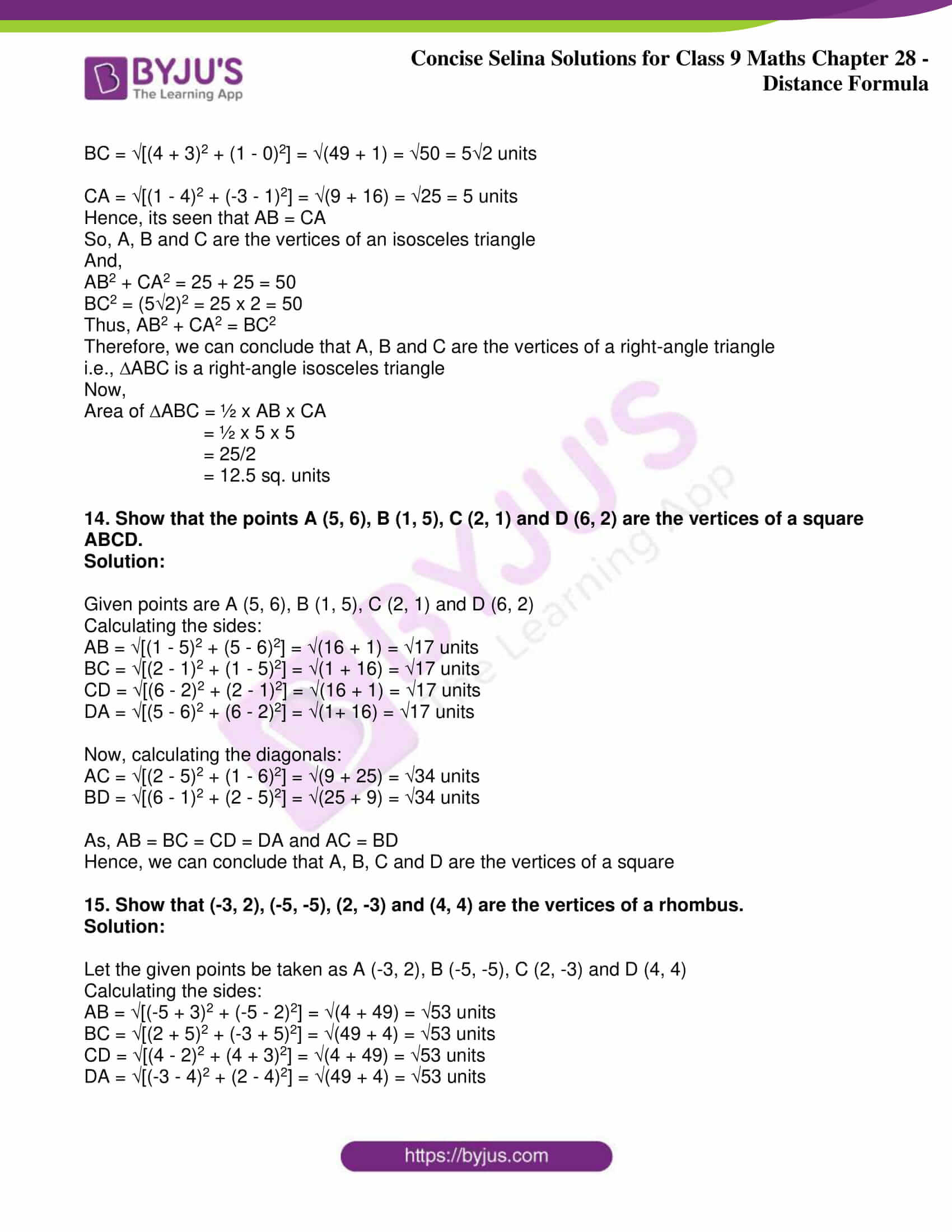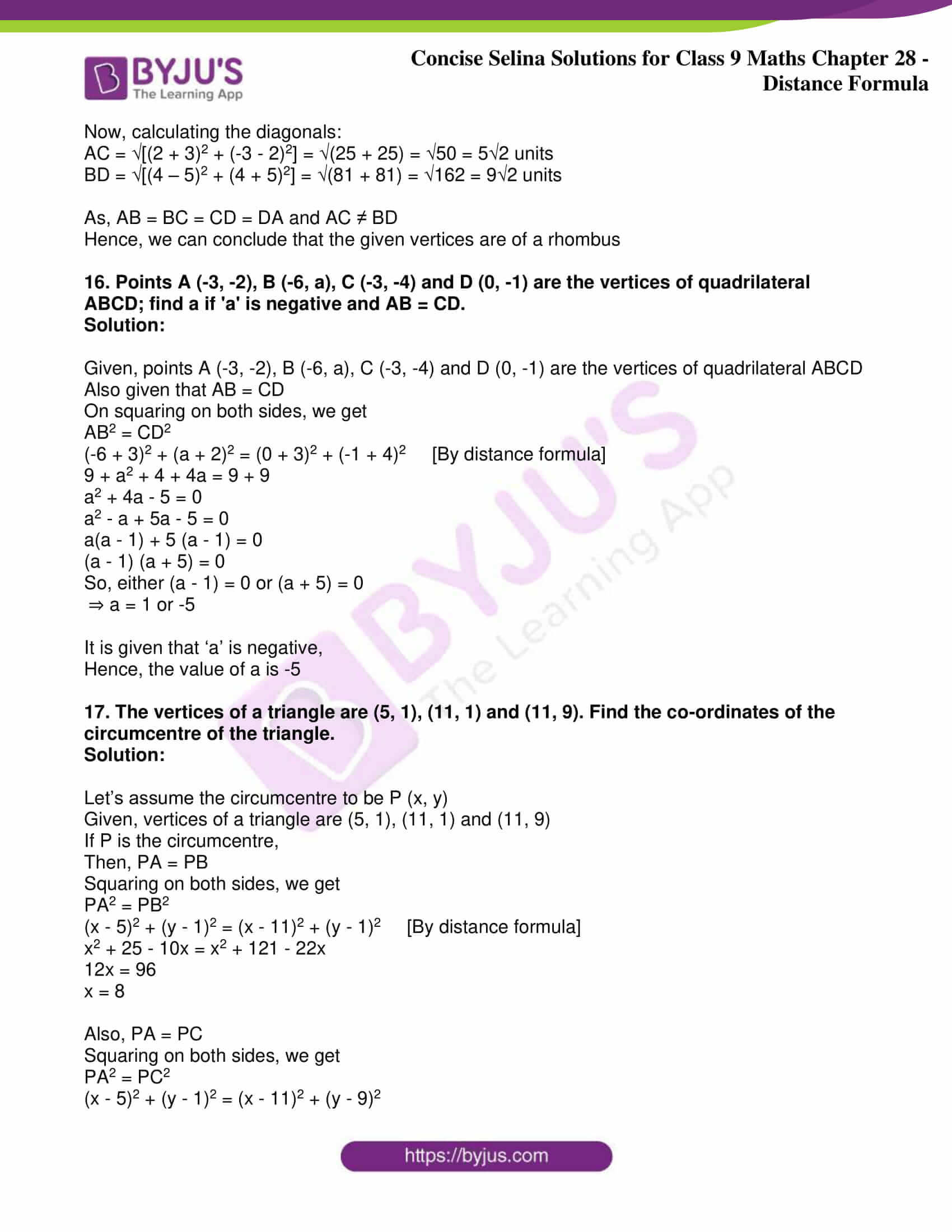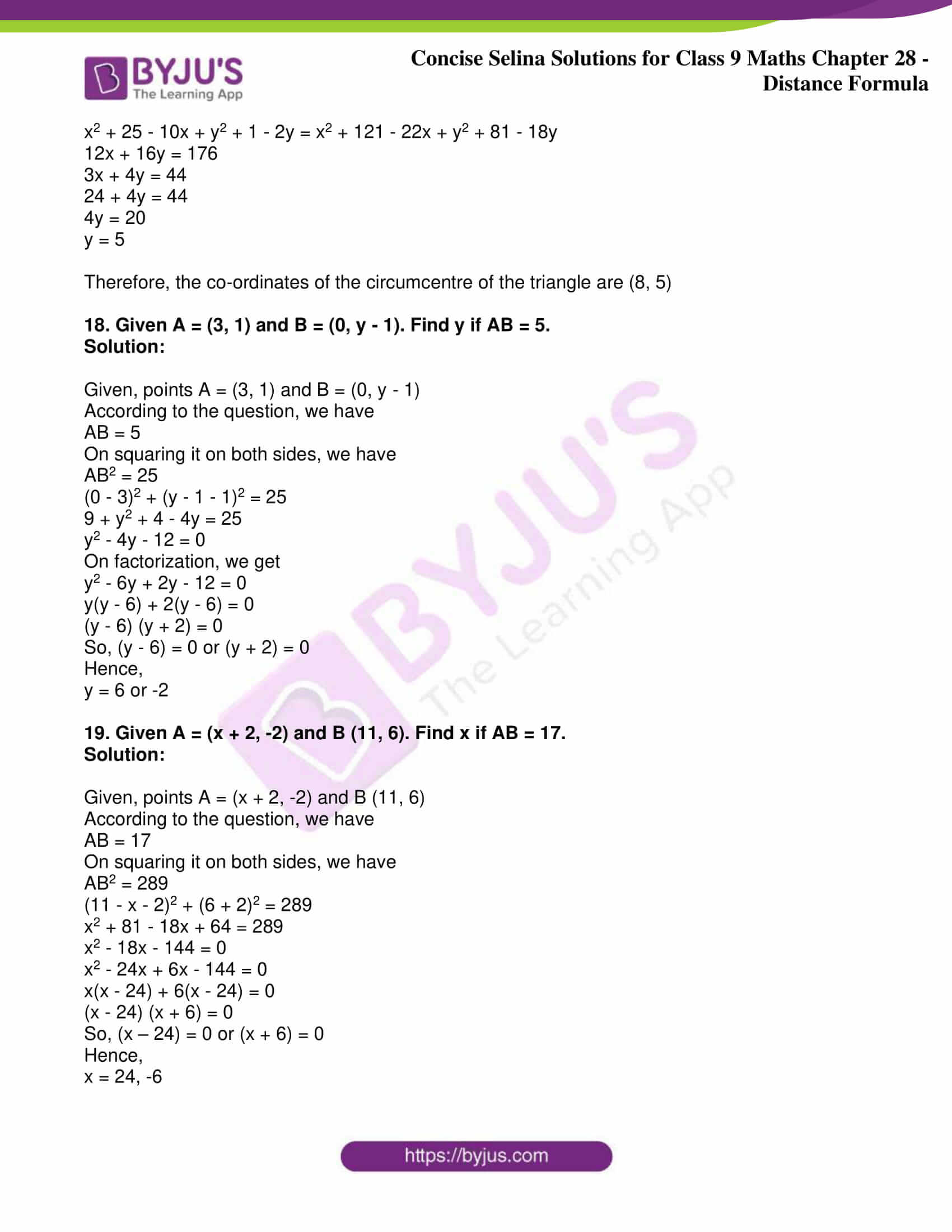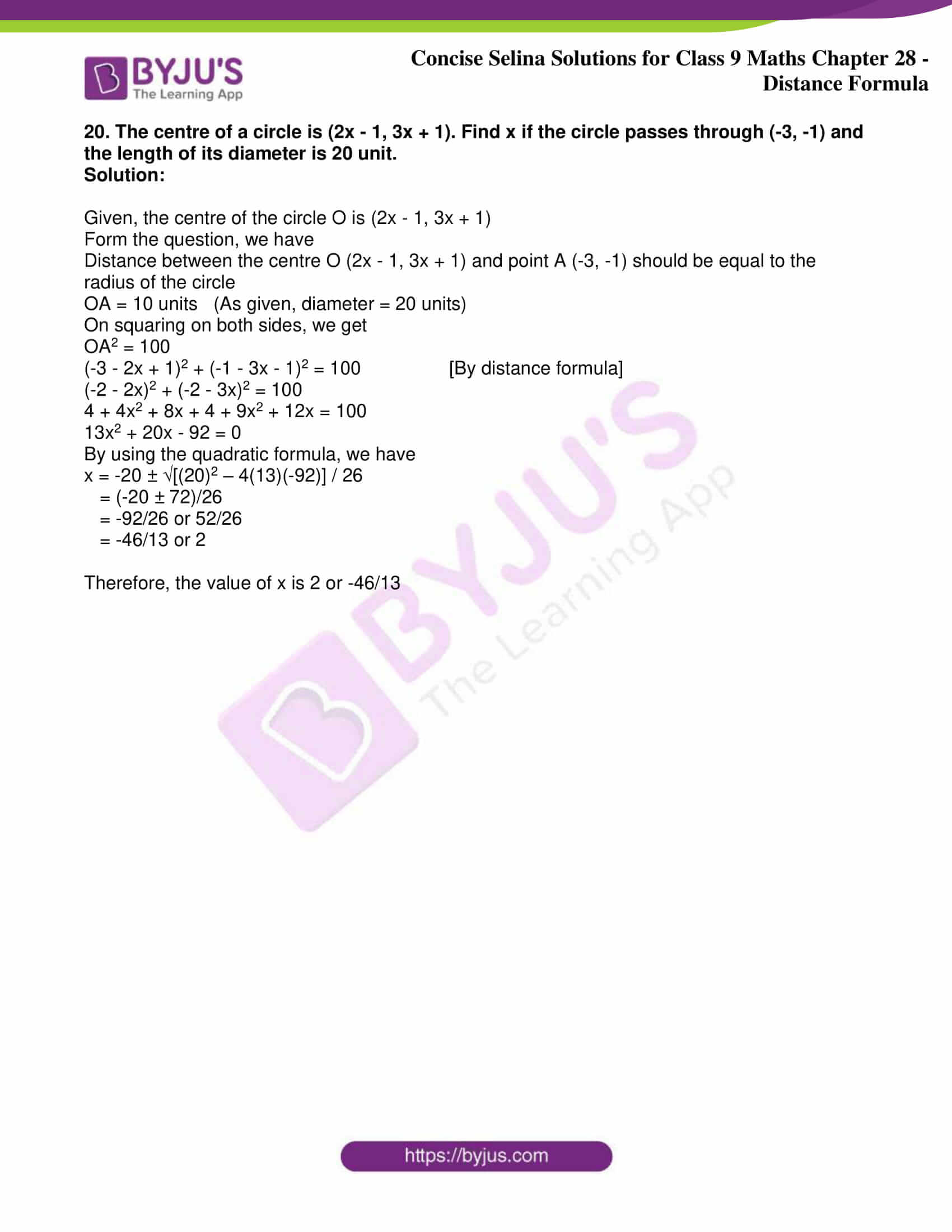### Access Answers to Maths Selina Problems of Class 9 Chapter 28- Distance Formula

Exercise 28

1. Find the distance between the following pairs of points:

(i) (-3, 6) and (2, -6)

(ii) (-a, -b) and (a, b)

(iii) (3/5, 2) and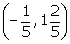(iv) (√3 + 1, 1) and (0, √3)

Solution:

(i) (-3, 6) and (2, -6)

Distance between the points is given by

= √[(2 -(-3))2 + (-6 – 6)2]

= √[52 + (-12)2]

= √(25 + 144)

= √169

= 13 units

(ii) (-a, -b) and (a, b)

Distance between the points is given by

= √[(a -(-a))2 + (b – (-b))2]

= √[(2a)2 + (2b)2]

= √(4a2 + 4b2)

= √4(a2 + b2)

= 2√(a2 + b2) units

(iii) (3/5, 2) andi.e., (3/5, 2) and (-1/5, 7/5)

Distance between the points is given by

= √[(-1/5 – 3/5)2 + (7/5 – 2)2]

= √[(-4/5)2 + ((7 – 10)/5)2]

= √(16/25 + 9/25)

= √(25/25)

= 1 unit

(iv) (√3 + 1, 1) and (0, √3)

Distance between the points is given by

= √[(0 -(√3 + 1))2 + (√3 – 1)2]

= √[(√3 + 1)2 + (√3 – 1)2]

= √(3 + 1 + 2√3 + 3 + 1 – 2√3)

= √8

= 2√2 units

2. Find the distance between the origin and the point:

(i) (-8, 6) (ii) (-5, -12) (iii) (8, -15)

Solution:

Coordinates of the origin O are (0, 0)

Now, the distance between the origin and the points are

(i) A (-8, 6)

AO = √[(0 + 8)2 + (0 – 6)2]

= √[82 + (-6)2]

= √(64 + 36)

= √100

= 10 units

(ii) B (-5, -12)

BO = √[(0 + 5)2 + (0 + 12)2]

= √[52 + 122]

= √(25 + 144)

= √169

= 13 units

(iii) C (8, -15)

CO = √[(0 – 8)2 + (0 + 15)2]

= √[82 + 152]

= √(64 + 225)

= √289

= 17 units

3. The distance between the points (3, 1) and (0, x) is 5. Find x.

Solution:

Given, the distance between the points A (3, 1) and B (0, x) is 5

AB = 5

On squaring on both sides, we get

AB2 = 52

By distance formula, we have

(0 – 3)2 + (x – 1)2 = 25

9 + x2 – 2x + 1 = 25

x2 – 2x + 10 = 25

x2 – 2x – 15 = 0

On factorization, we get

x2 + 3x – 5x – 15 = 0

x(x + 3) – 5(x + 3) = 0

(x – 5) (x + 3) = 0

So, either (x – 5) = 0 or (x + 3) = 0

Hence,

x = 5 or -3

4. Find the co-ordinates of points on the x-axis which are at a distance of 17 units from the point (11, -8).

Solution:

Let’s assume the coordinates of the point on x-axis to be (x, 0)

Now, from the question, we have

√[(x – 11)2 + (0 + 8)2] = 17 [By using distance formula]

On squaring on both sides, we get

(x – 11)2 + (0 + 8)2 = 289

x2 + 121 – 22x + 64 = 289

x2 – 22x – 104 = 0

On factorization, we get

x2 – 26x + 4x – 104 = 0

x(x – 26) + 4(x – 26) = 0

(x – 26) (x + 4) = 0

So, either (x – 26) = 0 or (x + 4) = 0

Hence,

x = 26 or -4

Therefore, the required co-ordinates of the points on x-axis are (26, 0) and (-4, 0)

5. Find the co-ordinates of the points on the y-axis, which are at a distance of 10 units from the point (-8, 4).

Solution:

Let’s assume the coordinates of the point on y-axis to be (0, y)

Now, from the question, we have

√[(0 + 8)2 + (y – 4)2] = 10 [By using distance formula]

On squaring on both sides, we get

82 + (y – 4)2 = 100

64 + y2 + 16 – 8y = 100

y2 – 8y – 20 = 0

On factorization, we get

y2 – 10y + 2y – 20 = 0

y(y – 10) + 2(y – 10) = 0

(y + 2) (y – 10) = 0

So, either (y + 2) = 0 or (y – 10) = 0

Hence,

y = 10 or -2

Therefore, the required co-ordinates of the points on y-axis are (0, 10) and (0, -2)

6. A point A is at a distance of √10 unit from the point (4, 3). Find the co-ordinates of point A, if its ordinate is twice its abscissa.

Solution:

Given, the co-ordinates of point A are such that its ordinate is twice its abscissa.

Now, let’s assume the co-ordinates of point A as (x, 2x)

And,

According to the question, we have

√[(x – 4)2 + (2x – 3)2] = √10 [By using distance formula]

On squaring on both sides, we get

(x – 4)2 + (2x – 3)2 = 10

x2 + 16 – 8x + 4x2 + 9 – 12x = 10

5x2 – 20x + 25 = 10

5x2 – 20x + 15 = 0

Dividing by 5, we get

x2 – 4x + 3 = 0

On factorization, we get

x2 – 3x – x + 3 = 0

x(x – 3) – (x – 3) = 0

(x – 3) (x – 1) = 0

So, either (x – 3) = 0 or (x -1) = 0

Hence,

x = 3 or 1

Thus, the co-ordinates of the point A are (1, 2) and (3, 6)

7. A point P (2, -1) is equidistant from the points (a, 7) and (-3, a). Find a.

Solution:

Given, the point P (2, -1) is equidistant from the points A (a, 7) and B (-3, a)

So, we have

PA = PB

⇒ PA2 = PB2

By using the distance formula, we have

(a – 2)2 + (7 + 1)2 = (-3 – 2)2 + (a + 1)2

a2 + 4 – 4a + 64 = 25 + a2 + 1 + 2a

68 – 4a = 26 + 2a

6a = 42

a = 7

Hence, the value of a is 7

8. What point on the x-axis is equidistant from the points (7, 6) and (-3, 4)?

Solution:

Let’s assume the co-ordinates of the required point on the x-axis to be P (x, 0)

The given points are A (7, 6) and B (-3, 4)

Given, PA = PB

So, on squaring on both sides, we get

PA2 = PB2

(x – 7)2 + (0 – 6)2 = (x + 3)2 + (0 – 4)2

x2 + 49 – 14x + 36 = x2 + 9 + 6x + 16

85 – 14x = 6x + 25

20x = 60

x = 3

Therefore, the required point is (3, 0)

9. Find a point on the y-axis which is equidistant from the points (5, 2) and (-4, 3).

Solution:

Let’s assume the co-ordinates of the required point on the y-axis to be P (0, y)

And. the given points are A (5, 2) and B (-4, 3)

Given, PA = PB

So, on squaring on both sides, we get

PA2 = PB2

(0 – 5)2 + (y – 2)2 = (0 + 4)2 + (y – 3)2

25 + y2 + 4 – 4y = 16 + y2 + 9 – 6y

29 – 4y = 25 – 6y

2y = -4

y = -2

Thus, the required point is (0, -2).

10. A point P lies on the x-axis and another point Q lies on the y-axis.

(i) Write the ordinate of point P.

(ii) Write the abscissa of point Q.

(iii) If the abscissa of point P is -12 and the ordinate of point Q is -16; calculate the length of line segment PQ.

Solution:

(i) As the point P lies on the x-axis, its ordinate will be 0

(ii) As the point Q lies on the y-axis, its abscissa will be 0

(iii) The co-ordinates of P and Q are (-12, 0) and (0, -16) respectively

And,

PQ = √[(-12 – 0)2 + (0 + 16)2]

= √(144 + 256)

= √400

= 20

11. Show that the points P (0, 5), Q (5, 10) and R (6, 3) are the vertices of an isosceles triangle.

Solution:

Given points are P (0, 5), Q (5, 10) and R (6, 3)

Calculating:

PQ = √[(5 – 0)2 + (10 – 5)2]

= √(25 + 25)

= √50

= 5√2

QR = √[(6 – 5)2 + (3 – 10)2]

= √(1 + 49)

= √50

= 5√2

RP = √[(0 – 6)2 + (5 – 3)2]

= √(36 + 4)

= √40

= 2√10

As PQ = QR

Hence, ∆PQR is an isosceles triangle.

12. Prove that the points P (0, -4), Q (6, 2), R (3, 5) and S (-3, -1) are the vertices of a rectangle PQRS.

Solution:

Given points are P (0, -4), Q (6, 2), R (3, 5) and S (-3, -1)

Calculating:

PQ = √[(6 – 0)2 + (2 + 4)2] = √(36 + 36) = √72 = 6√2 units

QR = √[(6 – 3)2 + (2 – 5)2] = √(9 + 9) = √18 = 3√2 units

RS = √[(3 + 3)2 + (5 + 1)2] = √(36 + 36) = √72 = 6√2 units

PS = √[(-3 – 0)2 + (-1 + 4)2] = √(9 + 9) = √18 = 3√2 units

PR = √[(3 – 0)2 + (5 + 4)2] = √(9 + 81) = √90 = 3√10 units

QS = √[(6 + 3)2 + (2 – 5)2] = √(9 + 9) = √18 = 3√2 units

It’s seen that,

PQ = RS and QR = PS

Also, PR = QS

Hence, PQRS is a rectangle

13. Prove that the points A (1, -3), B (-3, 0) and C (4, 1) are the vertices of an isosceles right-angled triangle. Find the area of the triangle.

Solution:

Given points are A (1, -3), B (-3, 0) and C (4, 1)

Calculating:

AB = √[(-3 – 1)2 + (0 + 3)2] = √(16 + 9) = √25 = 5 units

BC = √[(4 + 3)2 + (1 – 0)2] = √(49 + 1) = √50 = 5√2 units

CA = √[(1 – 4)2 + (-3 – 1)2] = √(9 + 16) = √25 = 5 units

Hence, its seen that AB = CA

So, A, B and C are the vertices of an isosceles triangle

And,

AB2 + CA2 = 25 + 25 = 50

BC2 = (5√2)2 = 25 x 2 = 50

Thus, AB2 + CA2 = BC2

Therefore, we can conclude that A, B and C are the vertices of a right-angle triangle

i.e., ∆ABC is a right-angle isosceles triangle

Now,

Area of ∆ABC = ½ x AB x CA

= ½ x 5 x 5

= 25/2

= 12.5 sq. units

14. Show that the points A (5, 6), B (1, 5), C (2, 1) and D (6, 2) are the vertices of a square ABCD.

Solution:

Given points are A (5, 6), B (1, 5), C (2, 1) and D (6, 2)

Calculating the sides:

AB = √[(1 – 5)2 + (5 – 6)2] = √(16 + 1) = √17 units

BC = √[(2 – 1)2 + (1 – 5)2] = √(1 + 16) = √17 units

CD = √[(6 – 2)2 + (2 – 1)2] = √(16 + 1) = √17 units

DA = √[(5 – 6)2 + (6 – 2)2] = √(1+ 16) = √17 units

Now, calculating the diagonals:

AC = √[(2 – 5)2 + (1 – 6)2] = √(9 + 25) = √34 units

BD = √[(6 – 1)2 + (2 – 5)2] = √(25 + 9) = √34 units

As, AB = BC = CD = DA and AC = BD

Hence, we can conclude that A, B, C and D are the vertices of a square

15. Show that (-3, 2), (-5, -5), (2, -3) and (4, 4) are the vertices of a rhombus.

Solution:

Let the given points be taken as A (-3, 2), B (-5, -5), C (2, -3) and D (4, 4)

Calculating the sides:

AB = √[(-5 + 3)2 + (-5 – 2)2] = √(4 + 49) = √53 units

BC = √[(2 + 5)2 + (-3 + 5)2] = √(49 + 4) = √53 units

CD = √[(4 – 2)2 + (4 + 3)2] = √(4 + 49) = √53 units

DA = √[(-3 – 4)2 + (2 – 4)2] = √(49 + 4) = √53 units

Now, calculating the diagonals:

AC = √[(2 + 3)2 + (-3 – 2)2] = √(25 + 25) = √50 = 5√2 units

BD = √[(4 – 5)2 + (4 + 5)2] = √(81 + 81) = √162 = 9√2 units

As, AB = BC = CD = DA and AC ≠ BD

Hence, we can conclude that the given vertices are of a rhombus

16. Points A (-3, -2), B (-6, a), C (-3, -4) and D (0, -1) are the vertices of quadrilateral ABCD; find a if ‘a’ is negative and AB = CD.

Solution:

Given, points A (-3, -2), B (-6, a), C (-3, -4) and D (0, -1) are the vertices of quadrilateral ABCD

Also given that AB = CD

On squaring on both sides, we get

AB2 = CD2

(-6 + 3)2 + (a + 2)2 = (0 + 3)2 + (-1 + 4)2 [By distance formula]

9 + a2 + 4 + 4a = 9 + 9

a2 + 4a – 5 = 0

a2 – a + 5a – 5 = 0

a(a – 1) + 5 (a – 1) = 0

(a – 1) (a + 5) = 0

So, either (a – 1) = 0 or (a + 5) = 0

⇒ a = 1 or -5

It is given that ‘a’ is negative,

Hence, the value of a is -5

17. The vertices of a triangle are (5, 1), (11, 1) and (11, 9). Find the co-ordinates of the circumcentre of the triangle.

Solution:

Let’s assume the circumcentre to be P (x, y)

Given, vertices of a triangle are (5, 1), (11, 1) and (11, 9)

If P is the circumcentre,

Then, PA = PB

Squaring on both sides, we get

PA2 = PB2

(x – 5)2 + (y – 1)2 = (x – 11)2 + (y – 1)2 [By distance formula]

x2 + 25 – 10x = x2 + 121 – 22x

12x = 96

x = 8

Also, PA = PC

Squaring on both sides, we get

PA2 = PC2

(x – 5)2 + (y – 1)2 = (x – 11)2 + (y – 9)2

x2 + 25 – 10x + y2 + 1 – 2y = x2 + 121 – 22x + y2 + 81 – 18y

12x + 16y = 176

3x + 4y = 44

24 + 4y = 44

4y = 20

y = 5

Therefore, the co-ordinates of the circumcentre of the triangle are (8, 5)

18. Given A = (3, 1) and B = (0, y – 1). Find y if AB = 5.

Solution:

Given, points A = (3, 1) and B = (0, y – 1)

According to the question, we have

AB = 5

On squaring it on both sides, we have

AB2 = 25

(0 – 3)2 + (y – 1 – 1)2 = 25

9 + y2 + 4 – 4y = 25

y2 – 4y – 12 = 0

On factorization, we get

y2 – 6y + 2y – 12 = 0

y(y – 6) + 2(y – 6) = 0

(y – 6) (y + 2) = 0

So, (y – 6) = 0 or (y + 2) = 0

Hence,

y = 6 or -2

19. Given A = (x + 2, -2) and B (11, 6). Find x if AB = 17.

Solution:

Given, points A = (x + 2, -2) and B (11, 6)

According to the question, we have

AB = 17

On squaring it on both sides, we have

AB2 = 289

(11 – x – 2)2 + (6 + 2)2 = 289

x2 + 81 – 18x + 64 = 289

x2 – 18x – 144 = 0

x2 – 24x + 6x – 144 = 0

x(x – 24) + 6(x – 24) = 0

(x – 24) (x + 6) = 0

So, (x – 24) = 0 or (x + 6) = 0

Hence,

x = 24, -6

20. The centre of a circle is (2x – 1, 3x + 1). Find x if the circle passes through (-3, -1) and the length of its diameter is 20 unit.

Solution:

Given, the centre of the circle O is (2x – 1, 3x + 1)

Form the question, we have

Distance between the centre O (2x – 1, 3x + 1) and point A (-3, -1) should be equal to the radius of the circle

OA = 10 units (As given, diameter = 20 units)

On squaring on both sides, we get

OA2 = 100

(-3 – 2x + 1)2 + (-1 – 3x – 1)2 = 100 [By distance formula]

(-2 – 2x)2 + (-2 – 3x)2 = 100

4 + 4x2 + 8x + 4 + 9x2 + 12x = 100

13x2 + 20x – 92 = 0

By using the quadratic formula, we have

x = -20 ± √[(20)2 – 4(13)(-92)] / 26

= (-20 ± 72)/26

= -92/26 or 52/26

= -46/13 or 2

Therefore, the value of x is 2 or -46/13

## Selina Solutions for Class 9 Maths Chapter 28- Distance Formula

Chapter 28, Distance Formula, consists of 1 exercise and the solutions given here contain answers to all the questions present in these exercises. Let us have a look at some of the topics that are being discussed in this chapter.

28.1 Introduction

28.2 The distance formula

28.3 Circumcentre of a triangle

## Selina Solutions for Class 9 Maths Chapter 28- Distance Formula

For any two known points in a co-ordinate plane, the knowledge of co-ordinate geometry may be used to find:

1. the distance between the given points
2. the co-ordinates of a point which divides the line joining the given points in a given ratio
3. the co-ordinates of the mid-point of the line segment joining the two given points,
4. equation of the straight line through the given points
5. equation of the perpendicular bisector of a line segment, etc.

Read and learn the Chapter 28 of Selina textbook to get to know more about Distance Formula. Learn the Selina Solutions for Class 9 effectively to attain excellent results in the examination.